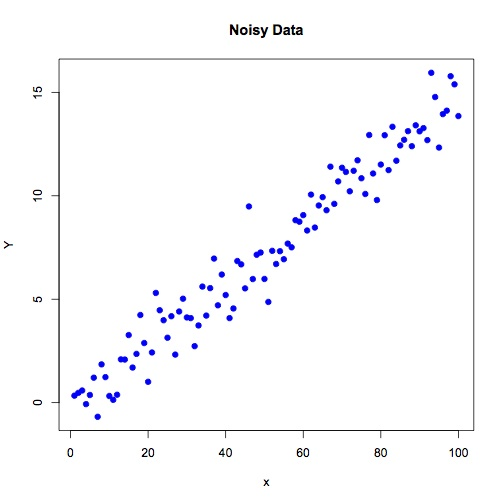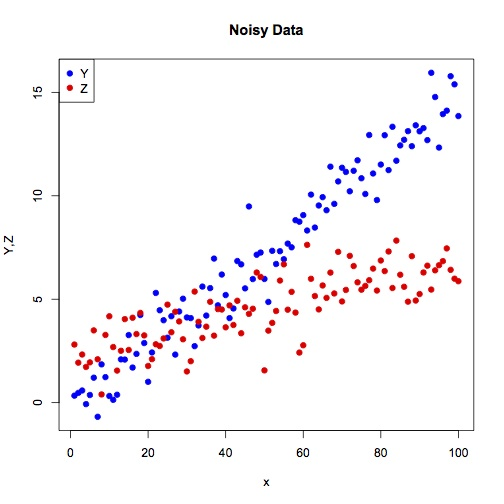# Exercise 22

## Scatterplot

Let x be a vector starting at 1 and ending at 100. Let y be a vector equal to (x * 0.15) + N where N is a vector of random values chosen from a gaussian distribution with mean 0.0 and standard deviation 1.0.

Generate a scatterplot of x vs y:(note your specific points might look different than mine, since there is random noise)

## A second variable

Let z be equal to ((x * 0.05) + 2) + N where N is a vector of random values chosen from a gaussian distribution with mean 0.0 and standard deviation 1.5.

Generate a scatterplot of y and z vs x, including a legend:Paul Gribble | fall 2014